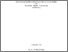Data adaptive inference for locally stationary processes

Richter, StefanPreview
PDF, English (Dissertation) - main document

Citation of documents: Please do not cite the URL that is displayed in your browser location input, instead use the DOI, URN or the persistent URL below, as we can guarantee their long-time accessibility.

Abstract

Since their introduction in the 1980s, locally stationary time series play an important role in time series analysis. As a generalization of stationary processes, they allow the observations to change their distribution properties over observation time. In many locally stationary time series models this change over time is characterized by parameter curves, whose estimation is of essential interest. In this work we develop methods and prove theoretical results for bandwidth selection for nonparametric estimators of these curves. We focus on local maximum likelihood estimators. Their strong connection to martingale difference sequences is fundamental in many of our proofs.

In the first part of this dissertation we define a global bandwidth selector for linear locally stationary processes which is motivated by the cross validation method that was first introduced in the nonparametric regression model. We prove that the selector is asymptotically optimal in the sense that the Kullback Leibler distance of the model connected with this selector to the true model converges to the minimal possible Kullback Leibler distance as the observation time increases to infinity. In simulations we analyze the quality of the method. The proofs are based on bias-variance decompositions of the estimators. The formal discussion of these decompositions gets harder in the case of nonlinear locally stationary time series models.

In the second part of this dissertation we develop general techniques to approximate locally stationary processes by stationary processes. These techniques allow us to obtain the decompositions mentioned above. We introduce so called derivative processes and give conditions under which existence and uniqueness can be guaranteed. An important result is a Taylor-like expansion of locally stationary processes. These findings are of independent interest for further research. We emphasize this point by using the approximation techniques to obtain new versions of standard theorems like a law of large numbers and a central limit theorem for locally stationary processes under minimal moment assumptions.

In the last part of this thesis we define a local bandwidth selector for a large class of locally stationary processes which is based on a contrast minimization approach which was first applied to nonparametric regression models. We show that our selector is minimax optimal up to a logarithmic factor (which is typical for local model selection procedures) with respect to the Euclidean distance and the Kullback-Leibler distance. For the proofs we use the approximation techniques which were discussed before. In a simulation we analyze the behavior of the selection routine for different time series models.

The findings of this thesis regarding bandwidth selection routines can be interpreted as a generalization of the original methods in the nonparametric regression model, because this model is included as a special case. Due to the more general formulation this thesis makes a contribution to understand these methods more deeply.

Item Type: Dissertation Dahlhaus, Prof. Dr. Rainer 6 December 2016 02 Jan 2017 14:41 2016 The Faculty of Mathematics and Computer Science > Department of Applied Mathematics 500 Natural sciences and mathematics510 Mathematics